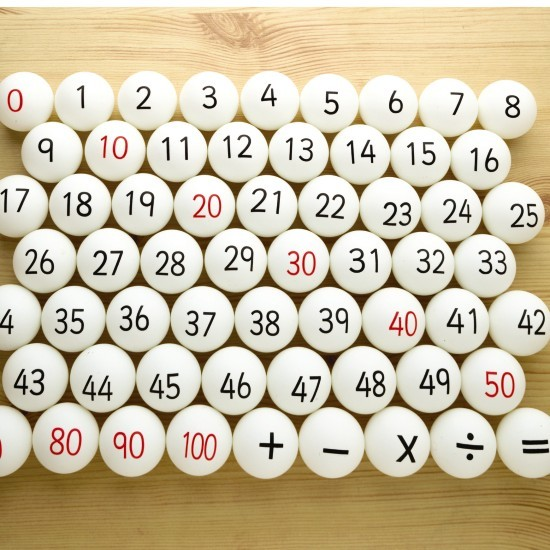# Will you accept the bet?

1,305.4K ViewsThere is a box in which distinct numbered balls have been kept. You have to pick two balls randomly from the lot.

If someone is offering you a 2 to 1 odds that the numbers will be relatively prime, for example
If the balls you picked had the numbers 6 and 13, you lose \$1.
If the balls you picked had the numbers 5 and 25, you win \$2.

Will you accept that bet?

Yes, you should accept the bet. Simply because the odds of picking two relatively prime numbers are 60%. It is a win-win situation for you if you keep playing.How do you know that the probability of picking two relatively prime numbers is 60%. Any proof or logic?

on 22nd September 2015.Relatively Prime – Two integers are relatively prime (or coprime) if there is no integer greater than one that divides them both

Proof- Probability of picking two relatively prime numbers is 60%

Think of this first
-What is the probability that a number randomly chosen will be divisible by 2?

-What about divisible by any prime p?
Its : 1/p

-What is the probability that two numbers chosen randomly are both divisible by prime p?
the events of the individual divisibilities are independent. therefore the answer must be 1/p^2.

-What if they are both not divisible by p?
1 – (1/p^2)

What if we involve another prime q here?
we have to multiply the values obtained then, right?
but the number of primes is infinite which means the product contains an infinitely many terms.

So,
we have mathematical tools to evaluate such stuff.
the product can be related to the evaluation of the Riemann zeta function at 2.
the answer comes out to be 6/π^2.
almost 61%.

Very good explaination at  – https://primes.utm.edu/notes/relprime.html

on 22nd September 2015.